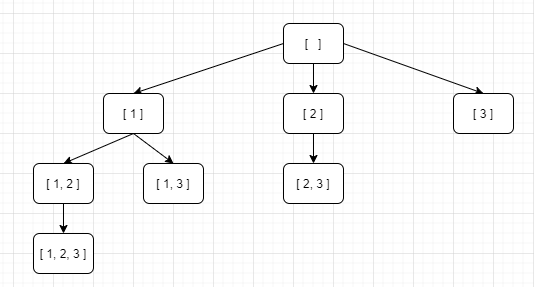# 题目

 `````` 1 2 3 4 5 6 7 8 9 10 11 12 `````` ``````输入: nums = [1,2,3] 输出: [ , , , [1,2,3], [1,3], [2,3], [1,2], [] ] ``````

# 题解`````` 1 2 3 4 5 6 7 8 9 10 11 12 13 14 15 16 17 18 19 `````` ``````vector> recurse(vector &nums) { vector> vv; for (int i = 0; i < nums.size(); i++) { vector copy(nums); copy.erase(copy.begin() + i); vv.emplace_back(copy); auto ret = recurse(copy); vv.insert(vv.begin(), ret.begin(), ret.end()); } return vv; } vector> subsets(vector& nums) { vector> vv; vv = recurse(nums); return vv; } ``````

 `````` 1 2 3 4 5 6 7 8 9 10 `````` ``````// 1的子集 []、 // 新增2的子集为 、[1,2] // 这样1、2的子集为 []、、、[1,2] // 新增3的子集为 、[1,3]、[2,3]、[1,2,3] // 1、2、3的子集为 []、、、[1,2]、、[1,3]、[2,3]、[1,2,3] ``````

 `````` 1 2 3 4 5 6 7 8 9 10 11 12 13 14 15 16 17 `````` ``````vector> subsets(vector& nums) { // base case，返回一个空集 if (nums.empty()) return {{}}; // 把最后一个元素拿出来 int n = nums.back(); nums.pop_back(); // 先递归算出前面元素的所有子集 vector> res = subsets(nums); int size = res.size(); for (int i = 0; i < size; i++) { // 然后在之前的结果之上追加 res.push_back(res[i]); res.back().push_back(n); } return res; } ```````````` 1 2 3 4 5 6 7 8 9 10 11 12 13 14 15 `````` ``````void recurse(vector &nums, int pos, vector track, vector> &vv) { vv.push_back(track); for (int i = pos; i < nums.size(); i++) { track.push_back(nums[i]); recurse(nums, i + 1, track, vv); track.pop_back(); } } vector> subsets(vector& nums) { vector> vv; vector v; recurse(nums, 0, v, vv); return vv; } ``````

000 []
001 
010 
011 [2,3]
100 
101 [1,3]
110 [1,2]
111 [1,2,3]

 `````` 1 2 3 4 5 6 7 8 9 10 11 12 13 `````` ``````class Solution: def subsets(self, nums: List[int]) -> List[List[int]]: n = len(nums) output = [] for i in range(2**n, 2**(n + 1)): # generate bitmask, from 0..00 to 1..11 bitmask = bin(i)[3:] # append subset corresponding to that bitmask output.append([nums[j] for j in range(n) if bitmask[j] == '1']) return output ``````